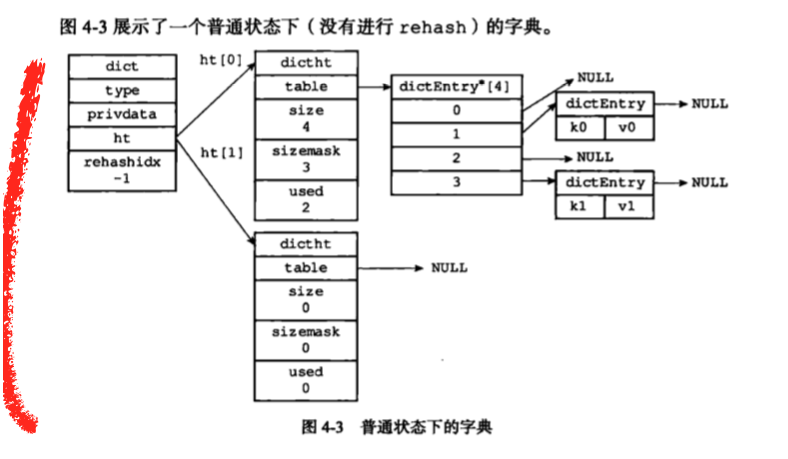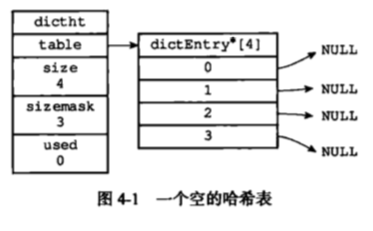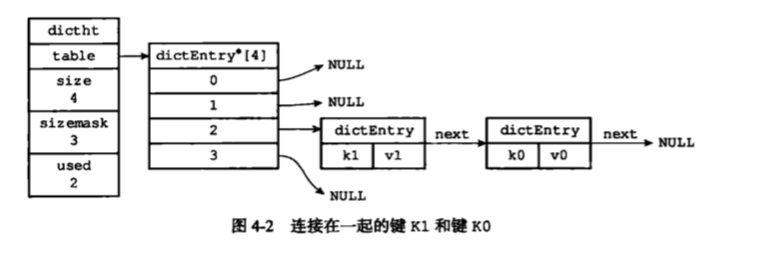scan遇上rehash

❐redis

# 背景

## keys和scan的区别

``````KEYS parttern：查找所有符合给定模式pattern的key。
``````
1. KEYS指令一次性返回所有匹配的key。
2. key的数量过大会使服务卡顿。
``````SCAN cursor [MATCH pattern] [COUNT count]：查找给定数量内个数的符合给定模式pattern的key。
``````
1. 基于游标的迭代器，需要基于上一次的游标延续之前的迭代过程。
2. 以0作为游标开始一次新的迭代，直到命令返回游标0完成一次遍历。
3. 不保证每次执行都返回给定数量的元素，支持模糊查询。
4. 一次返回的数量不可控，只能是大概率符合COUNT参数。
5. 返回的游标不一定是递增的，可能会获取到重复key，需要在外部程序去重。

## IO多路复用

1. fd拷贝（用户空间 -> 系统空间）
2. 遍历fd
3. 返回就绪的fd
4. 进程处理就绪fd请求

1. 没有limit，只能一次性的获取所有符合条件的结果，换句话说如果你的命令输入错误，可能会有成百上千的输出
2. 单进程单线程的redis，一个O(N)的算法，如果执行时间很长，很可能会直接导致redis服务的阻塞

# 问题

## dict结构``````dict结构
typedef struct dict {
//类型特定函数
dictType *type;
//私有数据
void *privData;
//哈希表
dictht ht;
//rehash索引
//当rehash不进行时，值为-1
int trehashidx;
}
``````

``````哈希表结构
typedef struct dictht {
//哈希表数组
dictEntry **table;
//哈希表大小
unsigned long size；
//哈希表大小掩码，用于计算索引值，总之等于size-1
//该哈希表已有节点的数量
unsigned long used;
}
``````

table是一个数组，数组中的每个元素都是指向dictEntry结构的指针，每个dictEntry保存这一个键值对``````hash节点结构
typedef struct dictEntry {
//键
void *key;
//值
union {
void *val;
uint64_tu64;
int64_ts64;
}v;
//指向下一个hash节点，形成链表
struct dictEntry *next;
} dictEntry;
``````## rehash导致的问题

``````//键值
string key = "USER_PHONE_123";
//根据键值计算hash值
hash_id = hashFunc(key);
//计算hash表掩码
//获取hash值对应的索引值
``````

``````000 -> 001 -> 010 -> 011 -> 100 -> 101 -> 110 -> 111 -> 000
0000 -> 0001 -> 0010 -> 0011 -> 0100 -> 0101 -> 0110 -> 0111 -> 1000 -> 1001 -> 1010 -> 1011 -> 1100 -> 1101 -> 1110 -> 1111 -> 0000

``````

# 解决

antirez: Hello @pietern! I’m starting to re-evaluate the idea of an iterator for Redis, and the first item in this task is definitely to understand better your pull request and implementation. I don’t understand exactly the implementation with the reversed bits counter… I wonder if there is a way to make that more intuitive… so investing some more time into this, and if I fail I’ll just merge your code trying to augment it with more comments… Hard to explain but awesome. pietern： Although I don’t have a formal proof for these guarantees, I’m reasonably confident they hold. I worked through every hash table state (stable, grow, shrink) and it appears to work everywhere by means of the reverse binary iteration (for lack of a better word).

## 反转二进制算法

``````unsigned long dictScan(dict *d,
unsigned long v,
dictScanFunction *fn,
void *privdata)
{
dictht *t0, *t1;
const dictEntry *de;
unsigned long m0, m1;

if (dictSize(d) == 0) return 0;

if (!dictIsRehashing(d)) {
t0 = &(d->ht);

de = t0->table[v & m0];
while (de) {
fn(privdata, de);
de = de->next;
}

} else {
t0 = &d->ht;
t1 = &d->ht;

if (t0->size > t1->size) {
t0 = &d->ht;
t1 = &d->ht;
}

de = t0->table[v & m0];
while (de) {
fn(privdata, de);
de = de->next;
}

do {
de = t1->table[v & m1];
while (de) {
fn(privdata, de);
de = de->next;
}

v = (((v | m0) + 1) & ~m0) | (v & m0);  // 就是对 v 的低 m1-m0 位加 1，并保留 v 的低 m0 位

} while (v & (m0 ^ m1));  // 循环条件 v &(m0 ^ m1)，表示直到 v 的低 m1-m0 位到低 m1 位之间全部为 0 为止。
}

v |= ~m0;

v = rev(v);
v++;
v = rev(v);

return v;
}
``````

``````#include <stdio.h>

// 对 v 进行二进制逆序操作
unsigned long rev(unsigned long v) {
unsigned long s = 8 * sizeof(v);
while ((s >>= 1) > 0) {
v = ((v >> s) & mask) | ((v << s) & ~mask);
}
return v;
}

//打印二进制
void printBits(unsigned long v, int tablesize)
{
int cnt = 0;
while(tablesize >> ++cnt);
for(int i = cnt-2; i >= 0; --i)
printf("%lu", (v >> i) & 1);
}

void test_dictScan_cursor(int tablesize)
{
unsigned long v;
unsigned long m0;

v = 0;
m0 = tablesize-1;
printBits(v, tablesize);

do
{
printf(" -> ");
//保留游标v的低m0位，其余位全为1
v |= ~m0;
//二进制进行翻转
v = rev(v);
//对二进制进行加1操作
v++;
//再次翻转
v = rev(v);
printBits(v, tablesize);
}while (v != 0);
printf("\n");
}

int main()
{
test_dictScan_cursor(8);
test_dictScan_cursor(16);
return 0;
}
``````

``````\$ gcc main.c -o main
\$ ./main
000 -> 100 -> 010 -> 110 -> 001 -> 101 -> 011 -> 111 -> 000
0000 -> 1000 -> 0100 -> 1100 -> 0010 -> 1010 -> 0110 -> 1110 -> 0001 -> 1001 -> 0101 -> 1101 -> 0011 -> 1011 -> 0111 -> 1111 -> 0000
``````